# Isosceles and Equilateral Triangles CONCEPT 25 1 Has

• Slides: 21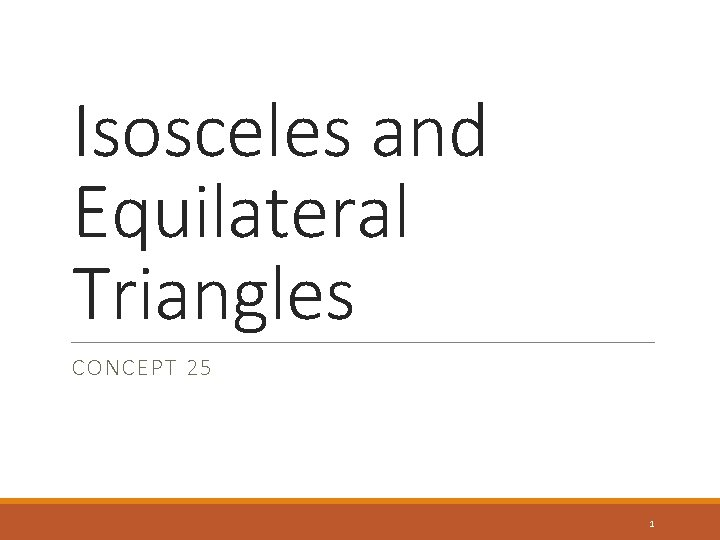Isosceles and Equilateral Triangles CONCEPT 25 1Has exactly three congruent sides Vertex Angle Leg the angle formed by the legs. the 2 congruent sides of an isosceles triangle. Base Angle 2 angles adjacent to the base. Base the 3 rd side of an isosceles triangle 23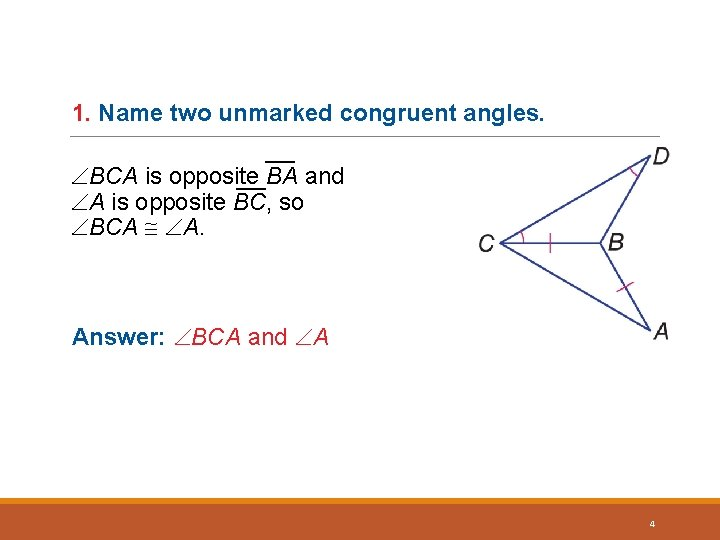1. Name two unmarked congruent angles. ___ BCA is opposite BA and ___ A is opposite BC, so BCA A. Answer: BCA and A 42. Name two unmarked congruent segments. ___ BC is opposite D and ___ BD is opposite BCD, so ___ BC BD. Answer: BC BD 5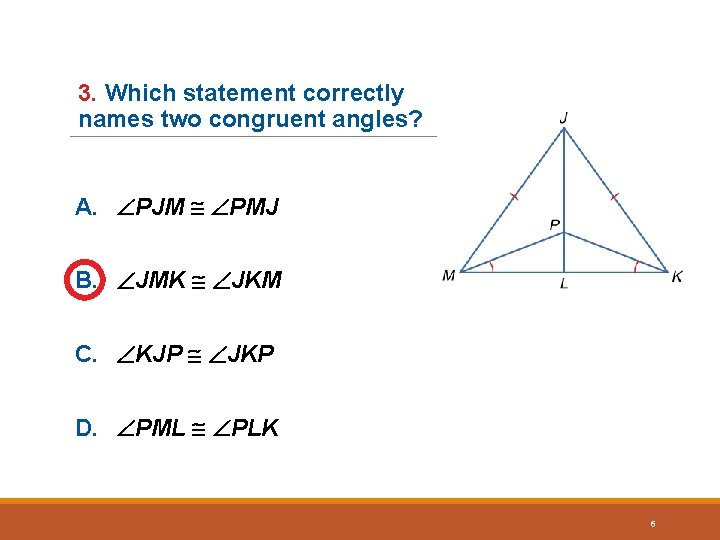3. Which statement correctly names two congruent angles? A. PJM PMJ B. JMK JKM C. KJP JKP D. PML PLK 6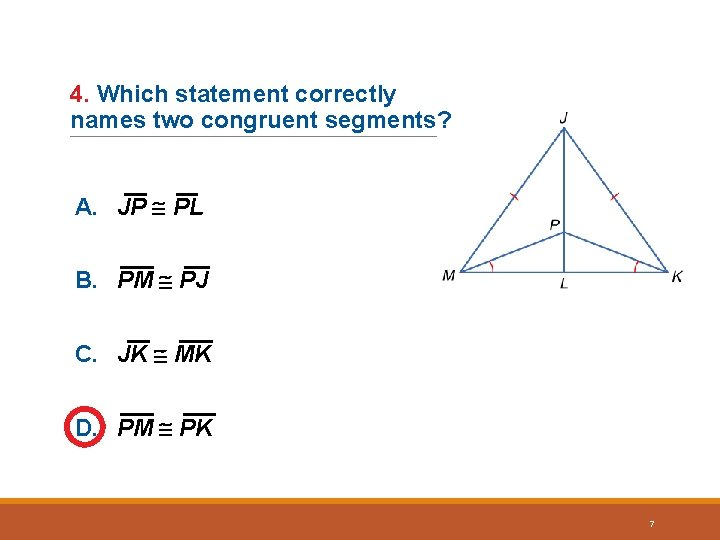4. Which statement correctly names two congruent segments? A. JP PL B. PM PJ C. JK MK D. PM PK 7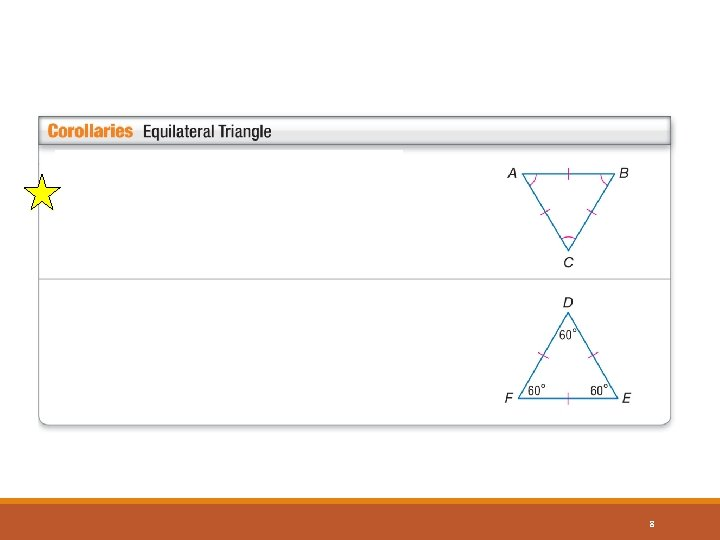8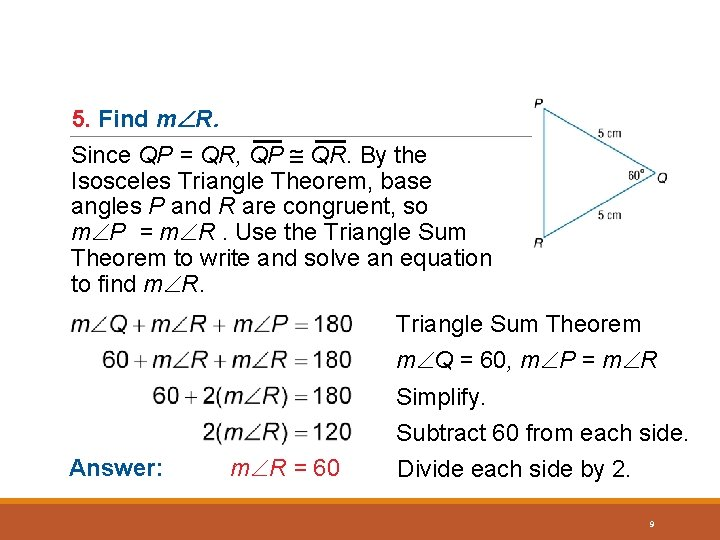5. Find m R. Since QP = QR, QP QR. By the Isosceles Triangle Theorem, base angles P and R are congruent, so m P = m R. Use the Triangle Sum Theorem to write and solve an equation to find m R. Triangle Sum Theorem m Q = 60, m P = m R Answer: m R = 60 Simplify. Subtract 60 from each side. Divide each side by 2. 9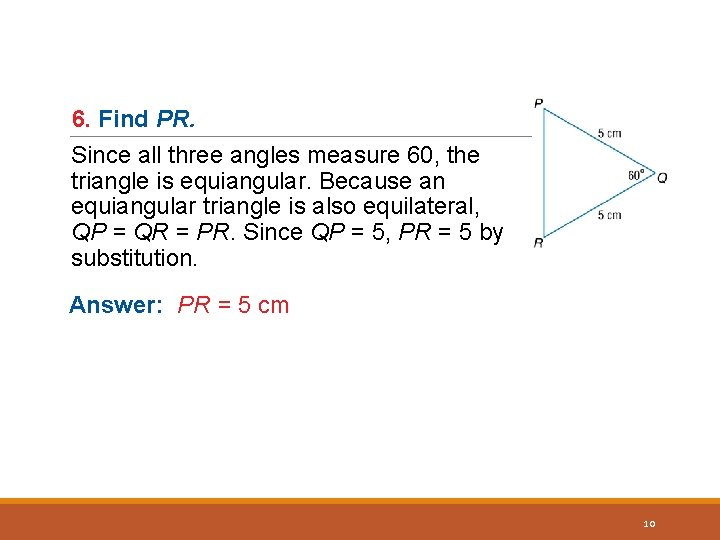6. Find PR. Since all three angles measure 60, the triangle is equiangular. Because an equiangular triangle is also equilateral, QP = QR = PR. Since QP = 5, PR = 5 by substitution. Answer: PR = 5 cm 10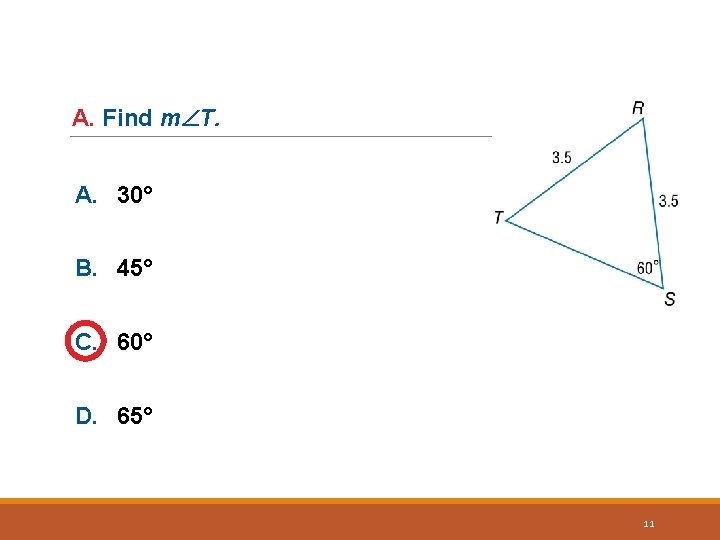A. Find m T. A. 30° B. 45° C. 60° D. 65° 11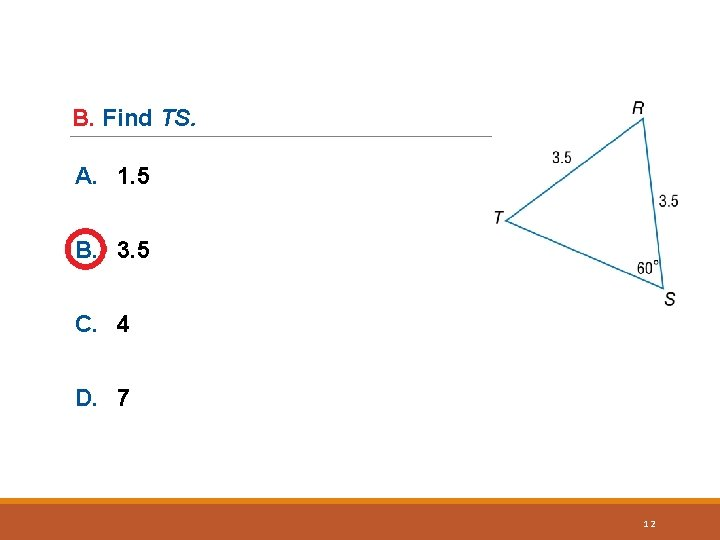B. Find TS. A. 1. 5 B. 3. 5 C. 4 D. 7 12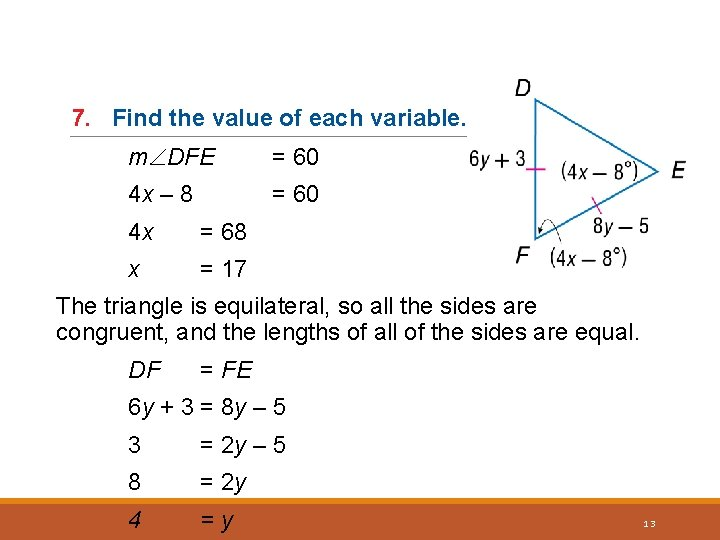7. Find the value of each variable. m DFE = 60 4 x – 8 = 60 4 x = 68 x = 17 The triangle is equilateral, so all the sides are congruent, and the lengths of all of the sides are equal. DF = FE 6 y + 3 = 8 y – 5 3 = 2 y – 5 8 = 2 y 4 =y 13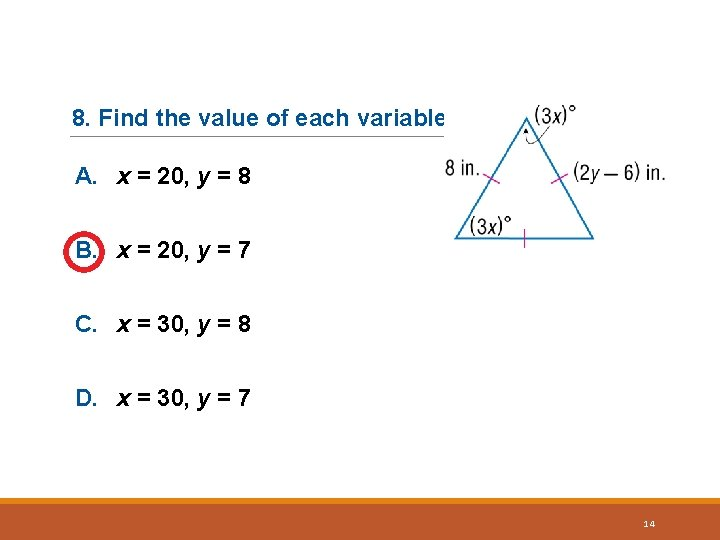8. Find the value of each variable. A. x = 20, y = 8 B. x = 20, y = 7 C. x = 30, y = 8 D. x = 30, y = 7 14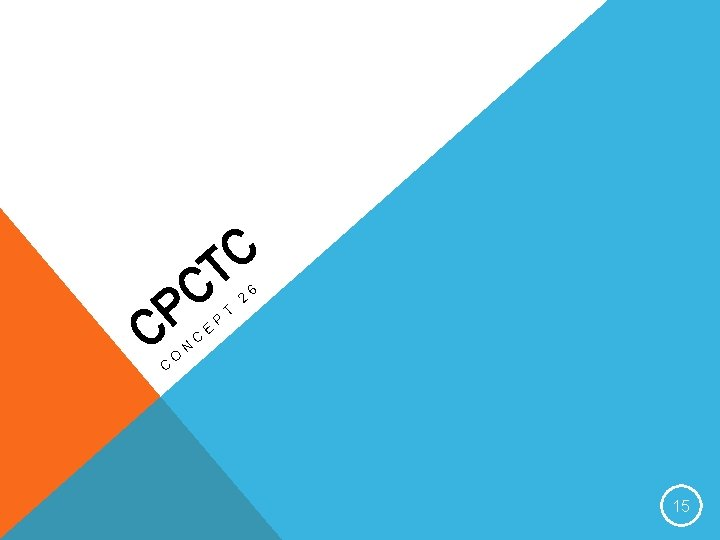C T C P C C O N C E P T 2 6 15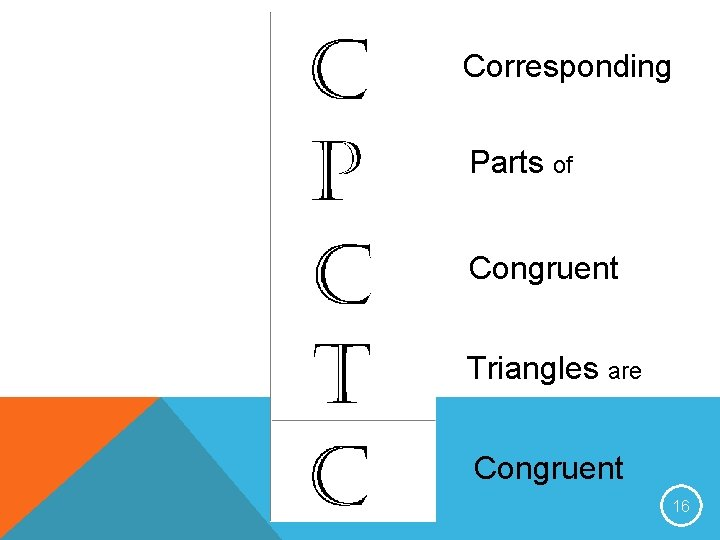Corresponding Parts of Congruent Triangles are Congruent 16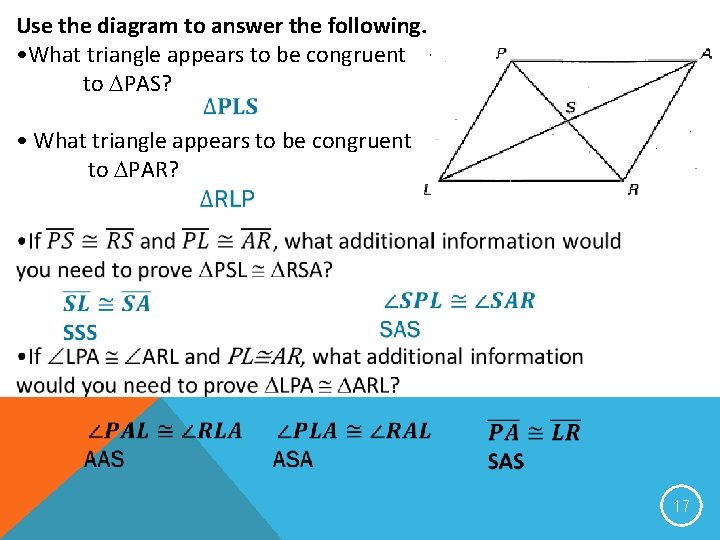Use the diagram to answer the following. • What triangle appears to be congruent to PAS? • What triangle appears to be congruent to PAR? 17Use the diagram to answer the following questions. LPS APS LRS ARS LPS ARS APS LRS 18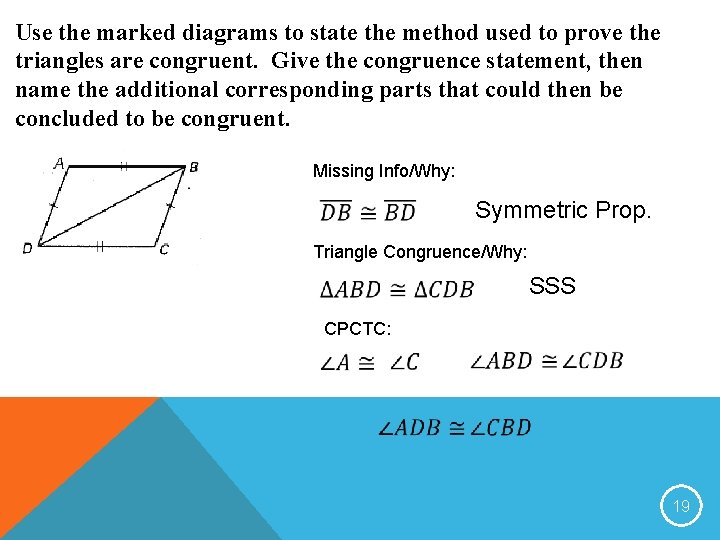Use the marked diagrams to state the method used to prove the triangles are congruent. Give the congruence statement, then name the additional corresponding parts that could then be concluded to be congruent. Missing Info/Why: Symmetric Prop. Triangle Congruence/Why: SSS CPCTC: 19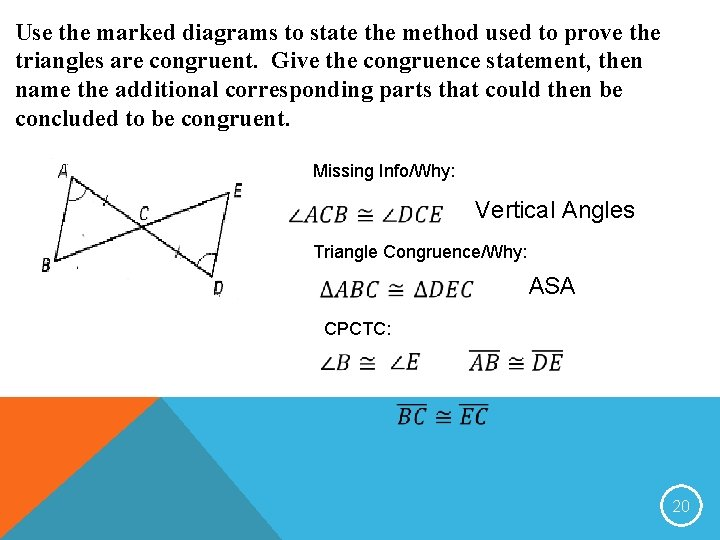Use the marked diagrams to state the method used to prove the triangles are congruent. Give the congruence statement, then name the additional corresponding parts that could then be concluded to be congruent. Missing Info/Why: Vertical Angles Triangle Congruence/Why: ASA CPCTC: 20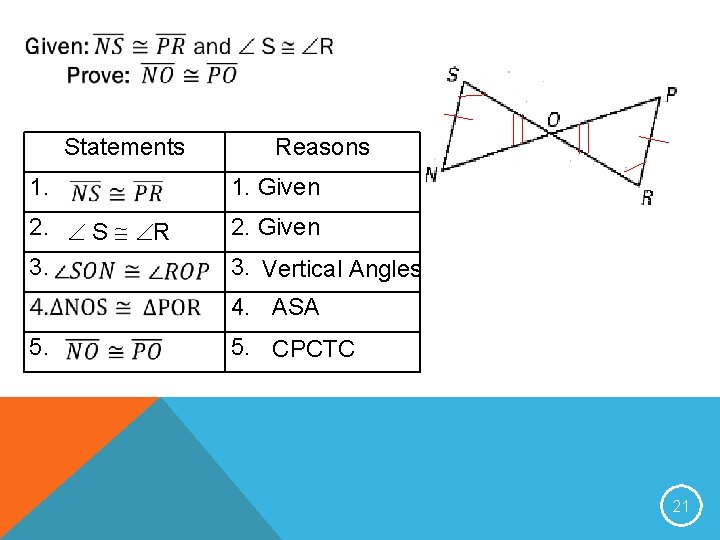Statements 1. Given 2. S R 3. 5. Reasons 2. Given 3. Vertical Angles 4. ASA 5. CPCTC 21